# Special relativity facts for kids

Kids Encyclopedia Facts
(Redirected from Special theory of relativity)

Special relativity (or the special theory of relativity) is a theory in physics that was developed and explained by Albert Einstein in 1905. It applies to all physical phenomena, so long as gravitation is not significant. Special relativity applies to Minkowski space, or "flat spacetime" (phenomena which are not influenced by gravitation).

Einstein knew that some weaknesses had been discovered in older physics. For example, older physics thought light moved in luminiferous aether. Various tiny effects were expected if this theory were true. Gradually it seemed these predictions were not going to work out.

Eventually, Einstein (1905) drew the conclusion that the concepts of space and time needed a fundamental revision. The result was special relativity theory. This is based on the constancy of the speed of light in all inertial frames of reference and the principle of relativity.

• inertial frame of reference: a frame of reference that describes time and space homogeneously, isotropically, and in a time-independent manner. Shorthand: space the same everywhere at all times.
• principle of relativity: the equations describing the laws of physics have the same form in all frames of reference. Shorthand: same equations work everywhere and at all times.

Galileo had already established the principle of relativity, which said that physical events must look the same to all observers, and no observer has the "right" way to look at the things studied by physics. For example, the Earth is moving very fast around the Sun, but we do not notice it because we are moving with the Earth at the same speed; therefore, from our point of view, the Earth is at rest. However, Galileo's math could not explain some things, such as the speed of light. According to him, the measured speed of light should be different for different speeds of the observer in comparison with its source. However, the Michelson-Morley experiment showed that this is not true, at least not for all cases. Einstein's theory of special relativity explained this among other things.

## Basics of special relativity

General relativity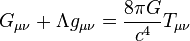$G_{\mu \nu} + \Lambda g_{\mu \nu}= {8\pi G\over c^4} T_{\mu \nu}$
Einstein field equations

Suppose that you are moving toward something that is moving toward you. If you measure its speed, it will seem to be moving faster than if you were not moving. Now suppose you are moving away from something that is moving toward you. If you measure its speed again, it will seem to be moving more slowly. This is the idea of "relative speed"—the speed of the object relative to you.

Before Albert Einstein, scientists were trying to measure the "relative speed" of light. They were doing this by measuring the speed of star light reaching the Earth. They expected that if the Earth was moving toward a star, the light from that star should seem faster than if the Earth was moving away from that star. However, they noticed that no matter who performed the experiments, where the experiments were performed, or what star light was used, the measured speed of light in a vacuum was always the same.

Einstein said this happens because there is something unexpected about length and duration, or how long something lasts. He thought that as the Earth moves through space, all measurable durations change very slightly. Any clock used to measure a duration will be wrong by exactly the right amount so that the speed of light remains the same. Imagining a "light clock" allows us to better understand this remarkable fact for the case of a single light wave.

Also, Einstein said that as the Earth moves through space, all measurable lengths change (ever so slightly). Any device measuring length will give a length off by exactly the right amount so that the speed of light remains the same.

The most difficult thing to understand is that events that appear to be simultaneous in one frame may not be simultaneous in another frame. This has many effects that are not easy to see or understand. Since the length of an object is the distance from head to tail at one simultaneous moment, it follows that if two observers disagree about what events are simultaneous then this will affect (sometimes dramatically) their measurements of the length of objects. Furthermore, if a line of clocks appear synchronized to a stationary observer and appear to be out of sync to that same observer after accelerating to a certain velocity then it follows that during the acceleration the clocks ran at different speeds. Some may even run backwards. This line of reasoning leads to general relativity.

Other scientists before Einstein had written about light seeming to go the same speed no matter how it was observed. What made Einstein's theory so revolutionary is that it considers the measurement of the speed of light to be constant by definition, in other words it is a law of nature. This has the remarkable implications that speed-related measurments, length and duration, change in order to accommodate this.

## The Lorentz transformations

The mathematical bases of special relativity are the Lorentz transformations, which mathematically describe the views of space and time for two observers who are moving relative to each other but are not experiencing acceleration.

To define the transformations we use a Cartesian coordinate system to mathematically describe the time and space of "events".
Each observer can describe an event as the position of something in space at a certain time, using coordinates (x,y,z,t).
The location of the event is defined in the first three coordinates (x,y,z) in relation to an arbitrary center (0,0,0) so that (3,3,3) is a diagonal going 3 units of distance (like meters or miles) out in each direction.
The time of the event is described with the fourth coordinate t in relation to an arbitrary (0) point in time in some unit of time (like seconds or hours or years).

Let there be an observer K who describes when events occur with a time coordinate t, and who describes where events occur with spatial coordinates x, y, and z. This is mathematically defining the first observer whose "point of view" will be our first reference.

Let us specify that the time of an event is given: by the time that it is observed t(observed) (say today, at 12 o'clock) minus the time that it took for the observation to reach the observer.

This can be calculated as the distance from the observer to the event d(observed) (say the event is on a star which is 1 light year away, so it takes the light 1 year to reach the observer) divided by c, the speed of light (several million miles per hour), which we define as being the same for all observers.
This is correct because distance, divided by speed gives the time it takes to go that distance at that speed (e.g. 30 miles divided by 10 mph: give us 3 hours, because if you go at 10 mph for 3 hours, you reach 30 miles). So we have: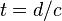$t = d/c$
This is mathematically defining what any "time" means for any observer.

Now with these definitions in place, let there be another observer K' who is

• moving along the x axis of K at a rate of v,
• has a spatial coordinate system of x' , y' , and z' ,

where x' axis is coincident with the x axis, and with the y' and z' axes - "always being parallel" to the y and z axes.

This means that when K', the second observer, gives a location like (3,1,2), the x (which is 3 in this example) is the same place that K, the first observer would be talking about, but the 1 on the y axis or the 2 on the z axis are only parallel to some location on the K' observer's coordinate system. and

• where K and K' are coincident at t = t' = 0
This means that the coordinate (0,0,0,0) is the same event for both observers.
In other words, both observers have (at least) one time and location that both agree on, which is location and time zero.

The Lorentz Transformations then are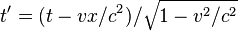$t' = (t - vx/c^2)/ \sqrt{1 - v^2/c^2}$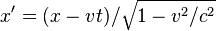$x' = (x - vt)/\sqrt{1 - v^2/c^2}$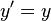$y' = y$, and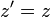$z' = z$.

## Mass, energy and momentum

In special relativity, the momentum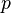$p$ and the total energy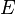$E$ of an object as a function of its mass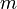$m$ are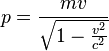$p = \frac {m v}{\sqrt{1 - \frac {v^2}{c^2}}}$

and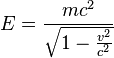$E = \frac {m c^2} {\sqrt{1 - \frac {v^2}{c^2}}}$.

A frequently made error (also in some books) is to rewrite these equation using a "relativistic mass" (in the direction of motion) of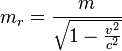$m_r =\frac {m} {\sqrt{1 - \frac {v^2}{c^2}}}$. The reason why this is incorrect is that light, for example, has no mass, but has energy. If we use this formula, the photon (particule of light) has a mass, which is according to experiments incorrect.

In special relativity, an object's mass, total energy and momentum are related by the equation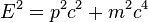$E^2 = p^2c^2 + m^2 c^4$.

For an object at rest,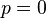$p = 0$ so the above equation simplifies to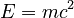$E = mc^2$. Hence, a massive object at rest still has energy. We call this rest energy and denote it by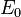$E_0$: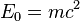$E_0 = mc^2$.

## History

The need for special relativity arose from Maxwell's equations of electromagnetism, which were published in 1865. It was later found that they call for electromagnetic waves (such as light) to move at a constant speed (i.e., the speed of light).

To have James Clerk Maxwell's equations be consistent with both astronomical observations, and Newtonian physics Maxwell proposed in 1877 that light travels through an ether which is everywhere in the universe.

In 1887, the famous Michelson-Morley experiment tried to detect the "ether wind" generated by the movement of the Earth. The persistent null results of this experiment puzzled physicists, and called the ether theory into question.

In 1895, Lorentz and Fitzgerald noted that the null result of the Michelson-Morley experiment could be explained by the ether wind contracting the experiment in the direction of motion of the ether. This effect is called the Lorentz contraction, and (without ether) is a consequence of special relativity.

In 1899, Lorentz first published the Lorentz equations. Although this was not the first time they had been published, this was the first time that they were used as an explanation of the Michelson-Morley experiment's null result, since the Lorentz contraction is a result of them.

In 1900, Poincaré gave a famous speech in which he considered the possibility that some "new physics" was needed to explain the Michelson-Morley experiment.

In 1904, Lorentz showed that electrical and magnetic fields can be modified into each other through the Lorentz transformations.

In 1905, Einstein published his article introducing special relativity, "On the Electrodynamics of Moving Bodies", in Annalen der Physik. In this article, he presented the postulates of relativity, derived the Lorentz transformations from them, and (unaware of Lorentz's 1904 article) also showed how the Lorentz Transformations affect electric and magnetic fields.

Later in 1905, Einstein published another article presenting E = mc2.

In 1908, Max Planck endorsed Einstein's theory and named it "relativity". In that same year, Minkowski gave a famous speech on Space and Time in which he showed that relativity is self-consistent and further developed the theory. These events forced the physics community to take relativity seriously. Relativity came to be more and more accepted after that.

In 1912 Einstein and Lorentz were nominated for the Nobel prize in physics due to their pioneering work on relativity. Unfortunately, relativity was so controversial then, and remained controversial for such a long time that a Nobel prize was never awarded for it.

## Experimental confirmations

• The Michelson-Morley experiment, which failed to detect any difference in the speed of light based on the direction of the light's movement.
• Fizeau's experiment, in which the index of refraction for light in moving water cannot be made to be less than 1. The observed results are explained by the relativistic rule for adding velocities.
• The energy and momentum of light obey the equation E = pc. (In Newtonian physics, this is expected to be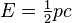$E = \begin{matrix} \frac{1}{2} \end{matrix} pc$.)
• The transverse doppler effect, which is where the light emitted by a quickly moving object is red-shifted due to time dilation.
• The presence of muons created in the upper atmosphere at the surface of the Earth. The issue is that it takes much longer than the half-life of the muons to get down to the surface of the Earth even at nearly the speed of light. Their presence can be seen as either being due to time dilation (in our view) or length contraction of the distance to the surface of the Earth (in the muon's view).
• Particle accelerators cannot be constructed without accounting for relativistic physics.

## Images for kidsSpecial relativity Facts for Kids. Kiddle Encyclopedia.